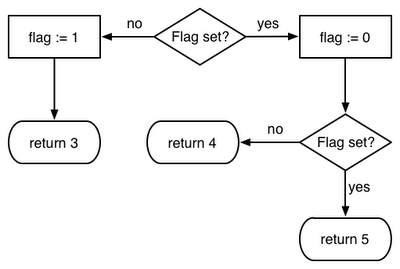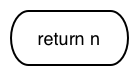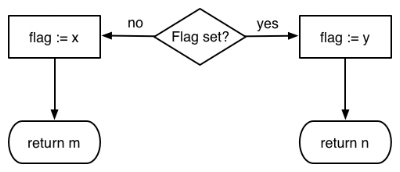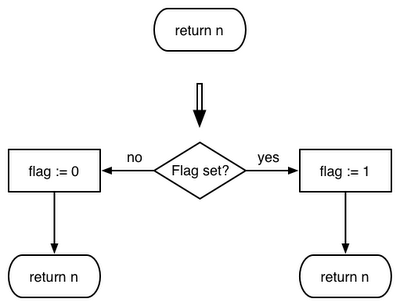## Saturday, December 05, 2009

### Where do monads come from?

Introduction
Monads play an important part in implementing computational "effects" in Haskell, and yet monads come out of pure mathematics where they are used to describe operations in algebraic structures. What do these two applications have to do with each other? In this post I hope to answer that question and maybe give another view on monads.

`> {-# LANGUAGE GADTs #-}`

Algebra for Flowcharts
You might not have seen one of these in a long time. A flowchart describing a subroutine:For now I'm considering flowcharts only with nodes to set or reset just one flag, test the flag, and return values. I'm not allowing any paths to reconverge. So these flowcharts are trees describing the possible paths of control flow.

We can describe such flowcharts with a more compact notation. Use xn to mean:Think of xn being an variable of unknown value.
Use the + operator to mean tests of the flag. So x+y represents:Note that this '+' isn't commutative because the left branch will always mean 'no' and the right branch will mean 'yes'.

And we'll use the symbols '0' and '1', applied on the left, to mean these two nodes respectively:The flowchart above can now be written compactly as 1x3+0(x4+x5). (Note that I'm overloading the notation for addition and multiplication, this isn't intended to be ordinary addition and multiplication of numbers.)

It's clear that the 'return 5' never gets executed. So the above flowchart is equivalent to this one:The rule describing this simplification can be expressed with our compact notation as the rule:

0(a+b) = 0a
1(a+b) = 1b

So we already have a strong clue that there is a connection between algebra and computation. Here are some more rules that you can check for yourself:

abc = bc (here a and b are each either 0 or 1)

a+(b+c) = a+c = (a+b)+c

a+a = a = 0a+1a

Substitution
Now suppose we wish to extend this subroutine so it does a bit more work. Instead of simply returning n we might want it to set a flag according to whether the previous result is even or odd, and subtract 1 if it is odd. It's a little awkward because I've insisted that these trees don't rejoin so we need to patch the tree in three places using the rule:Note that the descriptions of the two cases and the n here aren't part of the flowchart, they're part of the language I'm using to talk about flowcharts.

We can do something similar for our algebraic expressions. We can define a mathematical function taking integers to algebraic expressions such that

f(n)
= 0xn if n is even
= 1xn-1 if n is odd

and now define an operation * by

f*u = u with xn replaced by f(n) throughout

Our updated flowchart can now be represented by f*(1x3+0(x4+x5)). Now you might see why I used the weird xn notation. The * operation is ordinary substitution of an expression for a variable that we learn in high school, so I used xn notation to make it clear that xn is a variable. You can think of f as a dictionary saying how the values of the variables are to be expanded.

Let TN be the set of expressions in our algebra where the subscripts of the x's come from the set N. Then we have the functions:

η:N→TN defined by η(n)=xn
and
μ:T(T(N))→T(N) defined by μ(a)=i*a, where i is the identity.

We have a monad. (Exercise: check the monad laws.) Note that this definition isn't specific to our particular algebra. This definition applies to a very broad class of algebraic expression. Monads in mathematics are used to describe substitution of subexpressions into expressions. But I hope you can now see why they have a role to play in computing: the act of substituting an expression for a variable is essentially the same thing as grafting more operations on to the end of our tree of computations.

But if you look at the definitions of typical monads in the Haskell libraries, they don't look much like trees, and the implementation of >>= doesn't look much like grafting trees. The rest of this post will be about working through a specific example to show that it really is about tree grafting after all.

A Type for Expression Trees
We'll need to define a type to represent our algebraic expressions in Haskell. The n is going to be used to label our variables, and s will be explained shortly:

`> data Expr s n where`

Now we need constructors to represent 0 and 1. We can simplify this by combining them into one constructor and using the type Bool to signify whether it is 0 or 1 that we want. But there's no need to restrict ourselves to Bool so I'll make the code more general and use s instead:

`>    Put :: s -> Expr s n -> Expr s n`

So 0 is False and 1 is True and `Put` is our multiplication operator. (Maybe you've already guessed why I called it that.)

The next constructor will be for addition. I'm going to give it the weird name Get. The obvious thing to write would be:
`    Get :: Expr s n -> Expr s n -> Expr s n`

We could uncurry that instead and use
`    Get :: (Expr s n, Expr s n) -> Expr s n`

But we know that a pair (a, a) is the same as a function Bool -> a. So I'm going to actually use:

`>    Get :: (s -> Expr s n) -> Expr s n`

So instead of giving us a binary node in a tree, Get gives us a possible infinite s-ary node. But we'll be working with s = Bool for now.

Now we need variables. We'll just use:

`>    Var :: n -> Expr s n`

This is a recursive type so we can write a fold for it. If we'd been using generic library we'd get the fold for free but I'm giving it explicitly:

`> fold :: (s -> w -> w) -> ((s -> w) -> w) -> (n -> w) -> Expr s n -> w> fold put get var (Put s x) = put s (fold put get var x)> fold put get var (Get c) = get (\s -> fold put get var (c s))> fold put get var (Var n) = var n`

We can implement the monad for substitution:

`> instance Monad (Expr s) where>   return n = Var n>   x >>= f = fold Put Get f x where`

Note how the fold recursively replaces Get with Get, Put with Put and Var n with f n.

By now the game's up. If you read my post from last week then you know that I'm building something like the State monad. But although this monad has a similar interface, internally it's nothing like State. For one thing, Expr is a tree type and internally it could end up with an arbitrarily large number of nodes. How can we extract the familar State monad from this?

Canonical Form
Expressions in various algebraic languages can often be reduced to a canonical form. For example, we have disjunctive normal form for (a fragment of) logic. Expressions in our language above can be reduced to a canonical form. In the language of flowcharts, every one of our flowcharts can be reduced to something of the form:Algebraically the claim is that any expression reduces to something of the form au+bv. In the general case the items a and b are of type s and u and v can be represented by elements of n. So this is something of type ((s, n), (s, n)). Again we replace the pair by a function to get the type s -> (n, s). So we want a function to convert to canonical form:

`> canonical :: Expr s n -> (s -> (n, s))> canonical = fold put get var where`

We can get a return box into canonical form like this:Algebraically this is xn→0xn+1xn and we can implement it as

`>   var n = \s -> (n, s)`

Now we need to simplify the application of 0 or 1 to something already in canonical form. 0(au+bv) = 0au = au and 1(au+bv) = 1bv = bv. Essentially it just picks the first or second term in the sum according to whether we're multiplying on the left by 0 or 1. But that's exactly what the function type in s -> (n, s) does. So we can just write:

`>   put s c = \t -> c s`

Now we need to consider sums of two terms in canonical form. We can derive this just by looking at the sum of two sums: (p+q)+(r+s). This is just p+s. We get this by picking the left branch twice or the right branch twice, so we're just applying our function twice to the same thing:

`>   get c s = c s s`

Comparing with my last post you should see that canonical is what I called an interpreter. So the act of interpreting these tree expressions is one and the same thing as reducing them to a canonical form. This shouldn't be so surprising - executing a functional program is itself a process of reduction to a normal form. Here's an example of using the above reduction to get our flowchart executed:

`> test1 =>   Get \$ \c -> if c>       then Put False \$>       Get \$ \d -> if d>           then Var 5>           else Var 4>       else>           Put True \$>           Var 3> go1 = canonical test1 False`

canonical returns a different tree to the original tree. But we can easily map back to trees again:

`> inject :: (s -> (n,s)) -> Expr s n> inject f = Get \$ (\(n, s) -> Put s (Var n)) . f`

So the composition inject . canonical takes a tree and reduces it to canonical form keeping it as a tree.

We can view things like this: the State s n type is just the Expr s n type modulo an equivalence relation. The usual State type is a tree but this fact is obscured because we only work with a representative of each equivalence class, not entire trees. But the Monad instance is still simply tree grafting. We can prove this as follows:

`> data State s n = State { runState :: s -> (n, s) }> instance Monad (State s) where>   return n = State \$ canonical \$ Var n>   State f >>= g = State \$ canonical \$ inject f >>= (inject . runState . g)`

Note how the implementations consist solely of isomorphisms or wrapping/unwrapping. The type State is simply inheriting the Monad instance from the tree type. We can now put Put and Get into a form usable in the monad. Again, we're just wrapping and "canonicalising" near-trivial operations.

`> get = State \$ canonical (Get (\s -> Var s))> put n = State \$ canonical \$ Put n (Var ())> test2 = do>   c <- get>   if c>       then do>           put False>           d <- get>           if d>               then return 5>               else return 4>       else do>           put True>           return 3> go2 = runState test2 False`

Summary
Like in the previous post We're starting with signatures for functions. We can form an expression tree type. With the substitution operation this naturally becomes a monad.

We also specified equations for our functions. This leads to the idea of a reduced form for our expression trees. The state monad (and, in fact, most of the standard monads) are examples of reduced forms. If we implement canonical for an algebra, the monad follows for free. More precisely: given a signature and equations, the tree type and monad can be derived automatically. It seems tricky to derive the rules for reducing expressions to canonical form, but once you've done that the monad for the reduced form can be derived automatically.

The act of evaluating a monadic expression is the same as reducing an expression tree to its canonical form.

Various Thoughts
Given two algebraic theories with their own signatures and equations they can be combined together. One obvious way is to simply "freely" throw all of the signatures and equations together. This gives the "sum" of the two theories. If we additionally impose some new equations, a certain type of compatibility condition between the theories, then we get the product. So starting with two theories we get the monads for the two theories and the monad for the sum or product. This might provide an alternative approach to monad transformers for combining effects. (Actually, I'm doubtful of this plan for automatically combining effects because surprising things can happen, but I still think there's something useful to be extracted from the theory.)

Could this form the basis for more monad tutorials? Programmers from imperative, functional and other backgrounds are familiar with the ideas of trees and substitutions.

By the way, it's sometimes useful to implement a monad by building a tree first and then interpreting it separately. That's what I did a few posts ago and I found it much easier than deriving a complete monad in one go.

These trees are related to the diagrams I drew here.

You can repeat much of what I said above with all kinds of algebraic structures. My ICFP talk was on what you can do with the monad that arises from vector spaces and a spent a slide or two on the rules for reduction to canonical form.

An earlier post of mine on substitution.

You can interpret the canonical form of the expressions above as saying that when you have a bunch of switches (in certain types of code) you can replace them with just one. This is reminiscent of the old folk theorem about loops. In fact, the algebra I describe above is a lot like Kleene Algebra with Tests which can be used to prove that result.

This was all motivated by this post on Lambda the Ultimate. Some comments by Derek Elkins (that he's probably long forgotten) and pieces of this by Conor McBride helped me write the code.

I originally planned to say more about forming sums and products of theories but the code is going to need more work...

Update: Following one of the links I gave above I just found Unimo which is closely related.Unknown said...

Hello. Great post! Do you have any pointers to books or other literature that does more stuff like what you did here (bridging category theory with computer science applications)?

Thanks.

Monday, 07 December, 2009Unknown said...

I had attempted to explain similar things on my blog a long long time ago. But, your explanation is much clearer. And, I think mine was a bit wrong. Simplifying too much is an easy way to say incorrect things.

Thanks for taking the time to explain complex things in an easy and correct way. It takes time to write that kind of posts.

Monday, 07 December, 2009Eelis said...

I hope you'll forgive me, but I was somewhat disappointed by this article (though I admittedly didn't get very far).

It started off great with an intriguing title and introduction. But then, totally out of the blue and without any hint of relevance or direction, it goes into a most tedious detailed description of some obscure and boring flowchart language with seemingly arbitrary restrictions and simplification rules and substitution and whatnot.. What a complete turn-off! I lost all interest and never made it to the part about monads.

Tuesday, 08 December, 2009sigfpe said...

Eelis,

I tried to warn you in the first line of the post! :-)

Tuesday, 08 December, 2009Will said...

η:N→TN defined by η(n)=xn

Shouldn't this be

η:N→T(N) defined by η(n)=xn

or

η:N→T N defined by η(n)=xn

Anyway, I find most of your stuff interesting. Keep up the good blogging.

Tuesday, 08 December, 2009sigfpe said...

Will,

It seems to be quite common in category theory to write the application of a functor F to an object X as FX rather than F(X), though I admit I haven't been consistent.

Tuesday, 08 December, 2009sigfpe said...

Here's the same question (on p.14), and much the same answer, but with more general notation: Monads and Theories.

Tuesday, 08 December, 2009< Fefo /> said...

Hi ! I'm new at Haskell, why is it necessary to use the GADTs extension for the code in this post??

Wednesday, 09 December, 2009sigfpe said...

Fefo,

GADTs are used because of the way I've written Put and Get. I could have written them in non-GADT style but I wanted to emphasize that though they are constructors I'm interpreting them as functions that perform actions.

Wednesday, 09 December, 2009